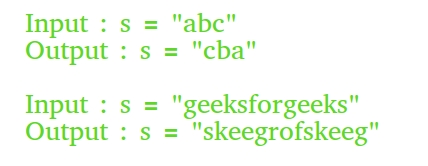Open In App

# Different Methods to Reverse a String in C++

The reversing of a string is nothing but simply substituting the last element of a string to the 1st position of the string.Different Methods to Reverse a String in C++ are:

• Making our own reverse function
• Using ‘inbuilt’ reverse function
• Using Constructor
• Using a temp file

### 1. Making a Custom Reverse Function For Swapping Characters

•  Using a first to last approach ‘for’ loop

## CPP

 `// C++ program to reverse a string``// using first to last approach``// 'for' loop``#include ``using` `namespace` `std;` `// Function to reverse a string``void` `reverseStr(string& str)``{``    ``int` `n = str.length();` `    ``// Swap character starting from two``    ``// corners``    ``for` `(``int` `i = 0; i < n / 2; i++)``        ``swap(str[i], str[n - i - 1]);``}` `// Driver program``int` `main()``{``    ``string str = ``"geeksforgeeks"``;``    ``reverseStr(str);``    ``cout << str;``    ``return` `0;``}`

Output

```skeegrofskeeg
```

Complexity Analysis:

Time Complexity: O(N)
Auxiliary Space: O(1)

• Using a first to last Approach with while loop

## C++

 `// C++ program to reverse a string``// using while loop``#include ``using` `namespace` `std;` `// Function to reverse a string``void` `reverseStr(string& str)``{``    ``int` `len = str.length();``    ``int` `n = len-1;``    ``int` `i = 0;``    ``while``(i<=n){``        ``//Using the swap method to switch values at each index``        ``swap(str[i],str[n]);``        ``n = n-1;``        ``i = i+1;``  ``}` `}` `// Driver program``int` `main()``{``    ``string str = ``"geeksforgeeks"``;``    ``reverseStr(str);``    ``cout << str;``    ``return` `0;``}`

Output

```skeegrofskeeg
```

Complexity Analysis:

Time Complexity: O(N)
Auxiliary Space: O(1)

•  Using a Last to First Approach ‘for‘ Loop

## C++

 `// C++ program to demonstrate reverse``// of a string using Last to First``// Approach 'for' Loop``#include ``using` `namespace` `std;` `// Function to reverse a string``void` `reverse(string str)``{``    ``for` `(``int` `i = str.length() - 1; i >= 0; i--)``        ``cout << str[i];``}` `// Driver code``int` `main(``void``)``{``    ``string s = ``"GeeksforGeeks"``;``    ``reverse(s);``    ``return` `(0);``}`

Output

```skeeGrofskeeG
```

Complexity Analysis:

Time Complexity: O(N)
Auxiliary Space: O(1)

• Using a Last to First Approach ‘while’ Loop

## C++

 `// C++ program to demonstrate reverse``// of a string using Last to First``// Approach 'while' Loop``#include ``using` `namespace` `std;` `// Function to reverse a string``void` `reverse(string str)``{``    ``int` `len = str.length();``    ``int` `n = len;``    ``while``(n--)``        ``cout << str[n];``}` `// Driver code``int` `main(``void``)``{``    ``string s = ``"GeeksforGeeks"``;` `    ``reverse(s);``    ``return` `(0);``}`

Output

```skeeGrofskeeG
```

Complexity Analysis:

Time Complexity: O(N)
Auxiliary Space: O(1)

### 1. Using recursion Function with two pointer approach

Recursion functions are used for iterating to different indexes of the string.

## C++

 `// C++ program to reverse a string``// using recursion``#include ``using` `namespace` `std;` `// Function to reverse a string``void` `reverseStr(string& str, ``int` `n, ``int` `i)``{``    ` `  ``if``(n<=i){``return``;}``//  Swapping the character``  ``swap(str[i],str[n]);``  ``reverseStr(str,n-1,i+1);` `}` `// Driver program``int` `main()``{``    ``string str = ``"geeksforgeeks"``;``    ``reverseStr(str, str.length()-1, 0);``    ``cout << str;``    ``return` `0;``}`

Output

```skeegrofskeeg
```

Complexity Analysis:

Time Complexity: O(N)

Auxiliary Space: O(N)

### 2. Using one pointer approach in recursion

Below is the implementation of the code:

## C++

 `//C++ program to reverse a string using recursion``#include ``using` `namespace` `std;``void` `getreverse(string &str, ``int` `i)``{``    ``if` `(i > (str.length() - 1 - i))``    ``{``        ``return``;``    ``}``    ``swap(str[i], str[str.length() - i - 1]);``    ``i++;``    ``getreverse(str, i);``}``int` `main()``{``    ``string name = ``"geeksforgeeks"``;` `    ``getreverse(name, 0);``    ``cout << name << endl;``    ``return` `0;``}``//code contributed by pragatikohli`

Output

```skeegrofskeeg

```

Complexity Analysis:

Time Complexity: O(N)

Auxiliary Space: O(N)

### 3. Using the inbuilt “reverse” Function

There is a direct function in the “algorithm” header file for doing reverse that saves our time when programming.

`// Reverses elements in [begin, end]void reverse (BidirectionalIterator begin, BidirectionalIterator end);`

## CPP

 `// C++ program to illustrate the``// reversing of a string using``// reverse() function``#include ``using` `namespace` `std;``int` `main()``{``    ``string str = ``"geeksforgeeks"``;` `    ``// Reverse str[begin..end]``    ``reverse(str.begin(), str.end());` `    ``cout << str;``    ``return` `0;``}`

Output

```skeegrofskeeg
```

Complexity Analysis:

Time Complexity: O(N)

Auxiliary Space: O(1)

### 4. Reverse a String Using the Constructor

Passing reverse iterators to the constructor returns us a reversed string.

## CPP

 `// C++ program to reverse``// string using constructor``#include ``using` `namespace` `std;``int` `main()``{``    ``string str = ``"GeeksforGeeks"``;` `    ``// Use of reverse iterators``    ``string rev = string(str.rbegin(), str.rend());` `    ``cout << rev << endl;``    ``return` `0;``}`

Output

```skeeGrofskeeG

```

Complexity Analysis:

Time Complexity: O(N)

Auxiliary Space: O(1)

## CPP

 `// C++ program to demonstrate``// reversing of string``// using temporary string``#include ``using` `namespace` `std;``int` `main()``{` `    ``string str = ``"GeeksforGeeks"``;``    ``int` `n = str.length();``  ` `    ``// Temporary string to store the reverse``    ``string rev;``  ` `    ``for` `(``int` `i = n - 1; i >= 0; i--)``        ``rev.push_back(str[i]);` `    ``cout << rev << endl;``    ``return` `0;``}`

Output

```skeeGrofskeeG

```

Complexity Analysis:

Time Complexity: O(N)

Auxiliary Space: O(1)

How could we get the reverse of a const string?

To get the reverse of a const string we have to first declare a ‘const string’ in a user-defined function following which we have declared then use the following algorithm for the calling of the desired objects.

`“const reverseConstString = function(string) { return string.split("").reverse().join("")” `

Example:

## C++

 `// C++ program to get reverse of a const string``#include ``using` `namespace` `std;` `// Function to reverse string and return``// reverse string pointer of that``char``* reverseConstString(``char` `const``* str)``{``    ``// find length of string``    ``int` `n = ``strlen``(str);` `    ``// create a dynamic pointer char array``    ``char``* rev = ``new` `char``[n + 1];` `    ``// copy of string to ptr array``    ``strcpy``(rev, str);` `    ``// Swap character starting from two``    ``// corners``    ``for` `(``int` `i = 0, j = n - 1; i < j; i++, j--)``        ``swap(rev[i], rev[j]);` `    ``// return pointer of the reversed string``    ``return` `rev;``}` `// Driver code``int` `main(``void``)``{``    ``const` `char``* s = ``"GeeksforGeeks"``;``    ``printf``(``"%s"``, reverseConstString(s));``    ``return` `(0);``}`

Output

```skeeGrofskeeG
```

Time Complexity: O(N)
Auxiliary Space: O(N)

Using Stack Data Structure

## C++

 `// C++ Program to reverse a string``#include ``using` `namespace` `std;``int` `main()``{``    ``string s = ``"GeeksforGeeks"``;``    ``stack<``char``> st;``    ``for` `(``char` `x : s)``        ``st.push(x);``    ``while` `(!st.empty()) {``        ``cout << st.top();``        ``st.pop();``    ``}``    ``return` `0;``}`

Output

```skeeGrofskeeG
```

Complexity Analysis:

Time Complexity: O(N)

Auxiliary Space: O(N)

Using Vector Data Structure

## C++

 `// C++ Program to reverse a string``#include ``using` `namespace` `std;``int` `main()``{``    ``string s = ``"GeeksforGeeks"``;``    ``int` `n=s.length();``    ``vector<``char``> vec;``    ``for` `(``int` `i = n - 1; i >= 0; i--)``        ``vec.push_back(s[i]);``    ` `    ``for``(``auto` `i:vec){``        ``cout<

Output

```skeeGrofskeeG
```

Complexity Analysis:

Time Complexity: O(N)

Auxiliary Space: O(N)